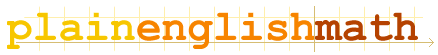You are here: MathematicsComplex Numbers → Introduction to Complex Numbers

# Introduction to Complex Numbers

## Imaginary numbers

Question: what is the square root of  negative one (often written as (-1)½ )?
For that matter, what is the square root of negative anything?
You may have been told that square roots of negative numbers are undefined; and equations involving them are impossible to solve. This is not strictly true. For example, lets say we define the letter i as follows:

Let  i = (-1)½

Now what is the square root of negative anything? For example, what is the square root of negative 49? Easy:

 (-49)½ = (49 × -1)½ = 49½ × (-1)½ = 7 × i = 7i

Of course, we haven't actually solved the square root - we've just buried the problem inside i. Numbers which are directly proportional to i, like i, 7i and bi (where b is a constant) are called imaginary numbers, and are technically meaningless. (All other numbers are called real numbers.)
So why bother with them at all? Because there are many instances where, in solving a difficult equation, terms involving square roots of negative numbers pop up. Introducing imaginary numbers allows us to proceed to the next step, and they often disappear from equations before the final solution anyway. For example, i2 = -1, thus higher order imaginary terms can 'decay' into real numbers.

Note that, likewise, all powers of i decay to either real or imaginary numbers of power 1. For example, consider i5:

 i5 = i2 i3 = -1 × i3 = -i2 i = -(-1)i = i

## Complex numbers

Now that you know what an imaginary number is, complex numbers are simple. A complex number is simply a real number plus an imaginary number. So there are in fact three types of numbers: real, imaginary and complex.

Complex numbers are of the form:

Z = a + bi

where a is the real component of complex number Z (often written as Re{Z}) and b = Im{Z} is the imaginary component.

Addition, multiplication, etc. of complex numbers is also simple. Just treat i exactly the same as any other algebraic symbol (x, y, a, b, etc.), and proceed as normal.